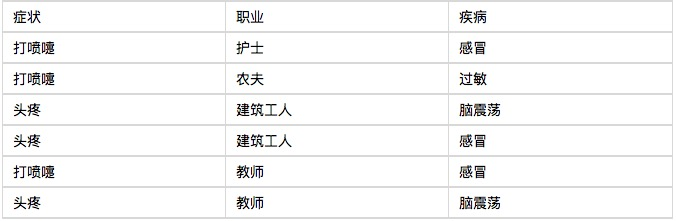# 趣味理解朴素贝叶斯

Author: 小小梦, Created: 2016-12-28 10:30:22, Updated: 2016-12-28 10:34:52

### 趣味理解朴素贝叶斯

#### NavieBayes

• 01 病人分类的例子

让我从一个例子开始讲起，你会看到贝叶斯分类器很好懂，一点都不难。某个医院早上收了六个门诊病人，如下表。现在又来了第七个病人，是一个打喷嚏的建筑工人。请问他患上感冒的概率有多大？ 根据贝叶斯定理：

``````P(A|B) = P(B|A) P(A) / P(B)
``````

可得：

``````P(感冒|打喷嚏x建筑工人)
``````

= P(打喷嚏x建筑工人|感冒) x P(感冒)  　　　　/ P(打喷嚏x建筑工人)

``````

``````

P(感冒|打喷嚏x建筑工人)  　　　　= P(打喷嚏|感冒) x P(建筑工人|感冒) x P(感冒) 　　　　/ P(打喷嚏) x P(建筑工人)

``````

``````

P(感冒|打喷嚏x建筑工人)  　　　　= 0.66 x 0.33 x 0.5 / 0.5 x 0.33  　　　　= 0.66

``````

- 02 朴素贝叶斯分类器的公式

``````

``````

``````

``````

``````

``````

#### 下面再通过两个例子，来看如何使用朴素贝叶斯分类器。

- 03 账号分类

C0 = 0.89
C1 = 0.11

F1: 日志数量/注册天数
F2: 好友数量/注册天数
F3: 是否使用真实头像（真实头像为1，非真实头像为0）
F1 = 0.1
F2 = 0.2
F3 = 0

P(F1|C)P(F2|C)P(F3|C)P(C)

P(F1|C0) = 0.5, P(F1|C1) = 0.1
P(F2|C0) = 0.7, P(F2|C1) = 0.2
P(F3|C0) = 0.2, P(F3|C1) = 0.9

P(F1|C0) P(F2|C0) P(F3|C0) P(C0)
= 0.5 x 0.7 x 0.2 x 0.89
= 0.0623
P(F1|C1) P(F2|C1) P(F3|C1) P(C1)
= 0.1 x 0.2 x 0.9 x 0.11
= 0.00198

- 04 性别分类

P(身高|性别) x P(体重|性别) x P(脚掌|性别) x P(性别)

P(身高=6|男) x P(体重=130|男) x P(脚掌=8|男) x P(男)

= 6.1984 x e-9

P(身高=6|女) x P(体重=130|女) x P(脚掌=8|女) x P(女)

= 5.3778 x e-4

#### 转载自 阮一峰 的微信公众号``````

More